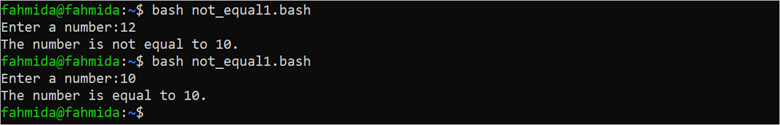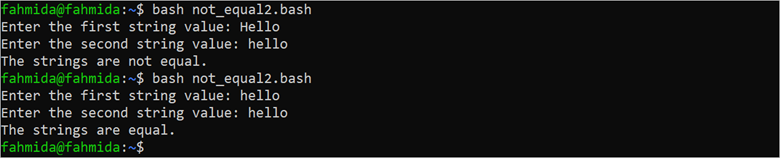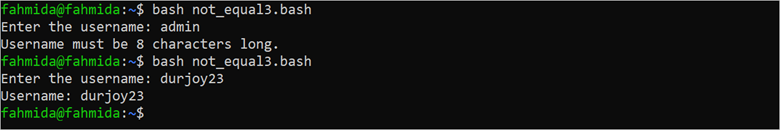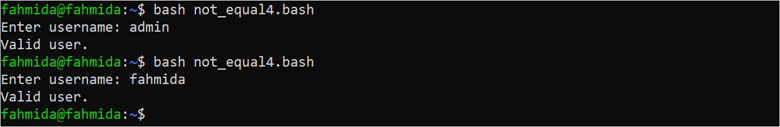| by Arround The Web | No comments

## Use of Not Equal to the Operator in Bash

Many types of operators exist in Bash to check the equality or inequality of two strings or numbers. The “-ne” and “!=” operators are used to check the inequality of two values in Bash. The single third brackets ([ ]) are used in the “if” condition when the “!=” operator is used to check the inequality. The double third brackets ([[ ]]) are used in the “if” condition when the “-ne” operator is used to check the inequality. The methods of comparing the string and numeric values using these operators are shown in this tutorial.

## Using the “!=” Operator

The “!=” operator can be used to check the inequality between two numeric values or two string values. Two uses of this operator are shown in the following examples.

Example 1: Checking the Inequality Between Numbers
Create a Bash file with the following script that takes a number input and check whether the input value is equal to 10 or not using the “!=” operator. The single third brackets ([ ]) are used in the “if” condition here.

#!/bin/bash
#Take a number
echo -n "Enter a number:"

#Use '!=' operator to check the number value
if [ \$number != 10 ]; then
echo "The number is not equal to 10."
else
echo "The number is equal to 10."
fi

The script is executed twice in the following output. Twelve (12) is taken as input in the first execution and “The number is not equal to 10” is printed. Ten (10) is taken as input in the second execution and “The number is equal to 10” is printed:Example 2:
Create a Bash file with the following script that takes two string values and check whether the input values are equal or not using the “!=” operator. The single third brackets ([ ]) are used in the “if” condition here.

#!/bin/bash
#Take a number
echo -n "Enter the first string value: "
echo -n "Enter the second string value: "

#Use '!=' operator to check the string values
if [ "\$str1" != "\$str2" ]; then
echo "The strings are not equal."
else
echo "The strings are equal."
fi

The script is executed twice in the following output. The “Hello” and “hello” string values are taken as inputs in the first execution and these values are not equal because the string values are compared case-sensitively. In the next execution, the “hello” and “hello” string values are taken as equal inputs:## Using the “-ne” Operator

The “-ne” operator can be used to check the inequality between two numeric values but not can be used to compare the string values. Two uses of this operator to compare the numeric and string values are shown in the following examples.

Example 1:
Create a Bash file with the following script that takes the username as input. Next, the length of the input value is counted after removing the newline(\n) character. Whether the length of the username is equal to 8 or not is checked using the “-ne” operator. The double third brackets ([[ ]]) are used in the “if” condition here.

#!/bin/bash

#Remove newline from the input value
#Count the total character

#Use the '-ne' operator to check the number value
if [[ \$len -ne 8 ]]; then
echo "Username must be 8 characters long."
else
fi

The script is executed twice in the following output. The “admin” is taken as input in the first execution and the “Username must be 8 characters long” is printed. The “durjoy23” is taken as input in the second execution and the “Username: durjoy23” is printed:Example 2:
Create a Bash file with the following script that takes the username as input. Next, whether the input value is equal to “admin” or not is checked using the “-ne” operator. The double third brackets ([[ ]]) are used in the “if” condition here. The “-ne” operator does not work to compare two string values.

#!/bin/bash

#Remove newline from the input value

#Use '-ne' operator to check the string values
echo "Invalid user."
else
echo "Valid user."
fi

The script is executed twice in the following output. The “if” condition is returned true in both executions for the valid and invalid outputs which is a “wrong” output:## Conclusion

The method of comparing two values using the “!=” and “-ne” operators are shown in this tutorial using multiple examples to know the uses of these operators properly.

Source: linuxhint.com